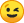• I began to expand beyond the border of Belgadevania. Warning that Belgadevania is not yet complete.Accursed Lands, a desertic biome formed by stones with a strange coloration at its pointed ends. "The ancients say that during the Invading God colonization, stones sprouted from the ground and there were blood in their pointed shapes".

I mix many objects, and this causes bugs in textures, so you need to modify the "config.properties" file: graphic_logarithmic_depthbuffer=true

• how do i "install" your preset of ReShade? I have ReShade up and working and in my RW-folder is a ReShade folder and 2 subfolders (Textures and Shaders).

• Theoretically you just need to create a preset and replace yours with mine.
I think you will want to reduce saturation, the colors are very strong in Accursed Land.

Effects=qUINT_lightroom.fx,FXAA.fx,AmbientLight.fx,SurfaceBlur.fx,ChromaticAberration.fx,Levels.fx,FakeHDR.fx,MagicBloom.fx,GaussianBlur.fx,qUINT_mxao.fx,qUINT_bloom.fx
KeyDepth3D=0,0,0,0
KeySmart_Sharp=0,0,0,0
Techniques=Lightroom,SurfaceBlur,AmbientLight,Levels,HDR,GaussianBlur,MagicBloom,FXAA,CA,MXAO,Bloom
KeyCross_Cursor=0,0,0,0
TechniqueSorting=Lightroom,SurfaceBlur,AmbientLight,Levels,HDR,GaussianBlur,MagicBloom,FXAA,CA,MXAO,Bloom,FilmGrain,KNearestNeighbors,RingDOF,NonLocalMeans,MagicDOF,MatsoDOF,GP65CJ042DOF,MartyMcFlyDOF,FilmGrain2,SMAA
KeyLightroom=79,0,0,0
KeyPolynomial_Barrel_Distortion_M=0,0,0,0

[SurfaceBlur.fx]
BlurOffset=0.820000
DebugMode=0
BlurEdge=2.200000
BlurStrength=1.000000

[Curves.fx]
Mode=2
Formula=4
Contrast=0.460000

[AmbientLight.fx]
alDebug=0
AL_DirtTex=0
alInt=4.000000
alThreshold=8.000000
AL_Dirt=1
alLensThresh=0.500000
AL_Vibrance=0
alDirtInt=1.000000
alLensInt=0.900000
alDirtOVInt=1.000000
AL_Lens=4294967295

[FXAA.fx]
Subpix=1.000000
EdgeThreshold=0.000000
EdgeThresholdMin=0.000000

[ReflectiveBumpMapping.fx]
fRBM_BlurWidthPixels=31.000000
iRBM_SampleCount=16
fRBM_LowerThreshold=0.020000
fRBM_ReliefHeight=0.660000
fRBM_FresnelReflectance=0.360000
fRBM_FresnelMult=0.300000
fRBM_UpperThreshold=0.200000

[Levels.fx]
BlackPoint=3
WhitePoint=190
HighlightClipping=0

[FilmicPass.fx]
Linearization=0.500000
Strength=0.850000
Contrast=1.000000
Bleach=0.000000
Saturation=-0.150000
GreenCurve=1.000000
RedCurve=1.000000
BlueCurve=1.000000
BaseCurve=1.500000
BaseGamma=1.000000
EffectGamma=0.650000
EffectGammaR=1.000000
EffectGammaG=1.000000
EffectGammaB=1.000000
LumCoeff=0.212656,0.715158,0.072186

[ChromaticAberration.fx]
Shift=-0.200000,3.600000
Strength=0.140000

[ColorMatrix.fx]
ColorMatrix_Red=0.800000,0.170000,0.200000
ColorMatrix_Green=0.293000,0.507000,0.170000
ColorMatrix_Blue=0.000000,0.000000,0.650000
Strength=0.440000

[Denoise.fx]
NoiseLevel=0.150000
LerpCoefficeint=0.800000
WeightThreshold=0.030000
CounterThreshold=0.050000
GaussianSigma=50.000000

[PerfectPerspective.fx]
FOV=45
Borders=4294967295
Vertical=0.000000
Type=0
Color=0.027000,0.027000,0.027000,0.902000
Zooming=1.076000

[qUINT_mxao.fx]
MXAO_GLOBAL_SAMPLE_QUALITY_PRESET=1
MXAO_DEBUG_VIEW_ENABLE=0
MXAO_SAMPLE_NORMAL_BIAS=0.272000
MXAO_GLOBAL_RENDER_SCALE=0.600000
MXAO_SSAO_AMOUNT=0.160000
MXAO_BLEND_TYPE=2

[LightDoF.fx]
fLightDoF_Width=5.000000
f2LightDoF_CA=0.000000,1.000000
f2Bokeh_AutoFocusCenter=0.500000,0.500000
fLightDoF_Amount=10.000000
bLightDoF_UseCA=0
bLightDoF_AutoFocus=1
fLightDoF_ManualFocus=0.000000
bLightDoF_UseMouseFocus=0
fLightDoF_AutoFocusSpeed=0.100000

[MXAO.fx]
MXAO_GLOBAL_SAMPLE_QUALITY_PRESET=2
MXAO_DEBUG_VIEW_ENABLE=0
MXAO_SAMPLE_NORMAL_BIAS=0.424000
MXAO_GLOBAL_RENDER_SCALE=0.740000
MXAO_SSAO_AMOUNT=0.120000
MXAO_BLEND_TYPE=0

[MagicBloom.fx]
fBloom_Intensity=1.000000
fBloom_Threshold=1.900000
fDirt_Intensity=0.074000
fExposure=0.059000
iDebug=0

[SMAA.fx]
EdgeDetectionType=1
EdgeDetectionThreshold=0.050000
MaxSearchSteps=83
MaxSearchStepsDiagonal=20
CornerRounding=100
DebugOutput=0

[Vibrance.fx]
Vibrance=-0.280000
VibranceRGBBalance=1.000000,1.000000,1.000000

[Tonemap.fx]
Gamma=0.960000
Bleach=1.000000
Defog=0.000000
Exposure=-0.620000
Saturation=-0.280000
FogColor=1.000000,1.000000,0.000000

[SuperDepth3D_v1.9.9.fx]
Auto_ZPD=2
Divergence=3.450000
Depth_Map=1
Depth_Map_Flip=0
Balance=6
Offsets=0.000000
ZPD=0.235000
Perspective=-30.000002
Depth_Map_View=0
WDM=15
Stereoscopic_Mode=0
Scaling_Support=1
Anaglyph_Colors=0
Anaglyph_Desaturation=1.000000
View_Mode=0
Auto_Depth_Range=0.000000
Eye_Swap=2147483648
InvertY=4294967295
Custom_Sidebars=1

[Polynomial_Barrel_Distortion_v1.3.fx]
Interpupillary_Distance=24.000000
Lens_Distortion=-0.325000,-0.325000
Stereoscopic_Mode_Convert=0.000000
Vertical_Repositioning=0.000000
Degrees=0.000000,0.000000
Lens_Center=0.500000
Polynomial_Colors=1.000000,1.000000,1.000000
Zoom_Aspect_Ratio=0.560000,1.165000
Custom_Sidebars=0.000000
Aliment_Marker=0.000000
Vignette=-2147483648.000000
Diaspora=-2147483648.000000
HMD_Profiles=0.000000

[Sepia.fx]
Tint=0.509804,0.498039,0.000000
Strength=0.160000

[Cartoon.fx]
Power=1.500000
EdgeSlope=1.500000

[Clarity.fx]
ClarityBlendMode=5
ClarityOffset=3.000000
ClarityDarkIntensity=0.610000
ClarityBlendIfDark=50
ClarityBlendIfLight=0
ClarityStrength=0.370000
ClarityLightIntensity=1.000000

[FakeHDR.fx]
HDRPower=2.260000

[FilmicAnamorphSharpen.fx]
Strength=1.000000
Offset=1
Coefficient=0
Clamp=1.000000
Contrast=128
Preview=0

[DOF.fx]
DOF_FARBLURCURVE=0.595000
DOF_AUTOFOCUS=4294967295
DOF_MOUSEDRIVEN_AF=0
fGPDOFChromaAmount=0.150000
DOF_INFINITEFOCUS=1.000000
fGPDOFBiasCurve=2.000000
DOF_FOCUSPOINT=0.000000,1.000000
DOF_FOCUSSAMPLES=6
DOF_MANUALFOCUSDEPTH=1.000000
DOF_NEARBLURCURVE=0.990000
fGPDOFBrightnessMultiplier=2.000000
bGPDOFPolygonalBokeh=1
fRingDOFFringe=0.500000
iRingDOFSamples=6
iRingDOFRings=4
fGPDOFBrightnessThreshold=0.500000
fRingDOFThreshold=0.700000
fRingDOFGain=14.153000
fRingDOFBias=0.000000
iMagicDOFBlurQuality=8
fMagicDOFColorCurve=4.000000
iGPDOFQuality=6
iGPDOFPolygonCount=5
fGPDOFBias=10.000000
bMatsoDOFChromaEnable=4294967295
fMatsoDOFChromaPow=1.572000
fMatsoDOFBokehCurve=6.545000
iMatsoDOFBokehQuality=3
fMatsoDOFBokehAngle=0.000000

[Colourfulness.fx]
lim_luma=1.000000
colourfulness=0.990000

[MultiLUT.fx]
fLUT_LutSelector=0
fLUT_AmountChroma=1.000000
fLUT_AmountLuma=1.000000

[Deband.fx]
Threshold=0.004000
Range=16.000000
Iterations=1
Grain=0.006000

[DepthHaze.fx]
EffectStrength=0.900000
FogStart=0.200000
FogColor=0.800000,0.800000,0.800000
FogFactor=0.200000

[3DFX.fx]
DITHERAMOUNT=0.500000
DITHERBIAS=-1
LEIFX_PIXELWIDTH=1.500000
LEIFX_LINES=1.000000
GAMMA_LEVEL=1.000000

[qUINT_lightroom.fx]
LIGHTROOM_GREEN_EXPOSURE=0.760000
LIGHTROOM_ENABLE_LUT=0
LIGHTROOM_LUT_TILE_SIZE=16
LIGHTROOM_LUT_TILE_COUNT=16
LIGHTROOM_MAGENTA_HUESHIFT=0.000000
LIGHTROOM_ENABLE_HISTOGRAM=0
LIGHTROOM_GLOBAL_TINT=0.000000
LIGHTROOM_LUT_SCROLL=0
LIGHTROOM_ENABLE_CURVE_DISPLAY=0
LIGHTROOM_BLUE_EXPOSURE=0.180000
LIGHTROOM_ENABLE_CLIPPING_DISPLAY=0
LIGHTROOM_GLOBAL_GAMMA=-0.060000
LIGHTROOM_HISTOGRAM_HEIGHT=0.000000
LIGHTROOM_GLOBAL_WHITES_CURVE=0.000000
LIGHTROOM_HISTOGRAM_SAMPLES=20
LIGHTROOM_MAGENTA_SATURATION=0.000000
LIGHTROOM_HISTOGRAM_SMOOTHNESS=5.000000
LIGHTROOM_RED_HUESHIFT=0.000000
LIGHTROOM_GLOBAL_BLACKS_CURVE=-0.280000
LIGHTROOM_GLOBAL_SATURATION=-0.580000
LIGHTROOM_ORANGE_HUESHIFT=0.000000
LIGHTROOM_GLOBAL_HIGHLIGHTS_CURVE=-0.700000
LIGHTROOM_YELLOW_HUESHIFT=0.000000
LIGHTROOM_GREEN_HUESHIFT=-0.240000
LIGHTROOM_AQUA_EXPOSURE=-0.180000
LIGHTROOM_AQUA_HUESHIFT=-0.240000
LIGHTROOM_BLUE_HUESHIFT=0.040000
LIGHTROOM_RED_EXPOSURE=0.000000
LIGHTROOM_ORANGE_EXPOSURE=0.620000
LIGHTROOM_YELLOW_EXPOSURE=0.880000
LIGHTROOM_MAGENTA_EXPOSURE=0.000000
LIGHTROOM_RED_SATURATION=0.220000
LIGHTROOM_ORANGE_SATURATION=0.000000
LIGHTROOM_YELLOW_SATURATION=0.000000
LIGHTROOM_GREEN_SATURATION=-0.560000
LIGHTROOM_AQUA_SATURATION=-0.120000
LIGHTROOM_BLUE_SATURATION=-0.140000
LIGHTROOM_GLOBAL_BLACK_LEVEL=4.000000
LIGHTROOM_VIGNETTE_CURVE=1.000000
LIGHTROOM_GLOBAL_WHITE_LEVEL=262.000000
LIGHTROOM_GLOBAL_EXPOSURE=-0.300000
LIGHTROOM_GLOBAL_MIDTONES_CURVE=0.000000
LIGHTROOM_GLOBAL_CONTRAST=-0.700000
LIGHTROOM_GLOBAL_VIBRANCE=0.660000
LIGHTROOM_GLOBAL_TEMPERATURE=0.080000
LIGHTROOM_ENABLE_VIGNETTE=0
LIGHTROOM_VIGNETTE_WIDTH=0.000000
LIGHTROOM_VIGNETTE_HEIGHT=0.000000
LIGHTROOM_VIGNETTE_AMOUNT=1.000000
LIGHTROOM_VIGNETTE_BLEND_MODE=1

[Bloom.fx]
bGodrayEnable=0
iBloomMixmode=2
fBloomSaturation=0.900000
bAnamFlareEnable=0
fBloomThreshold=0.856000
fLensdirtSaturation=2.000000
fBloomAmount=0.700000
fLensdirtIntensity=0.400000
fBloomTint=0.741176,0.717647,0.207843
fFlareLuminance=0.095000
bLensdirtEnable=4294967295
iLensdirtMixmode=1
bLenzEnable=0
fLensdirtTint=1.000000,1.000000,1.000000
fAnamFlareThreshold=0.900000
fAnamFlareWideness=2.400000
fAnamFlareAmount=14.500000
fAnamFlareCurve=1.200000
fAnamFlareColor=0.012000,0.313000,0.588000
fLenzIntensity=1.000000
fLenzThreshold=0.800000
bChapFlareEnable=0
fChapFlareTreshold=0.900000
iChapFlareCount=15
fChapFlareDispersal=0.250000
fFlareIntensity=2.070000
fChapFlareSize=0.450000
fChapFlareCA=0.000000,0.010000,0.020000
fChapFlareIntensity=100.000000
fGodrayDecay=0.990000
fGodrayExposure=1.000000
fGodrayWeight=1.250000
fGodrayDensity=1.000000
fGodrayThreshold=0.900000
iGodraySamples=128
fFlareBlur=200.000000
fFlareTint=0.137000,0.216000,1.000000

[CRT.fx]
ScanlineGaussian=1
Amount=1.000000
Resolution=1.150000
Curvature=0
Gamma=2.400000
Brightness=0.900000
MonitorGamma=2.200000
ScanlineIntensity=2
CornerSize=0.010000
ViewerDistance=2.000000
Angle=0.000000,0.000000
Overscan=1.010000
Oversample=1

[Emphasize.fx]
ManualFocusDepth=0.026000
FocusRangeDepth=0.010000
EffectFactor=0.900000
BlendColor=0.000000,0.000000,0.000000
FocusEdgeDepth=0.050000
BlendFactor=0.000000

[FilmGrain.fx]
Intensity=0.070000
Variance=0.890000
Mean=0.500000
SignalToNoiseRatio=3

[FilmGrain2.fx]
grainamount=0.050000
coloramount=0.600000
lumamount=1.000000
grainsize=1.600000

[GaussianBlur.fx]
GaussianBlurOffset=1.000000
GaussianBlurStrength=0.300000

FogCurve=1.500000
FogColor=0.900000,0.900000,0.900000
MaxFogFactor=0.800000
BloomPower=10.000000
FogStart=0.050000
BloomThreshold=10.250000
BloomWidth=0.200000

[HighPassSharpen.fx]
HighPassSharpOffset=1.000000
HighPassBlendMode=1
HighPassBlendIfLight=255
HighPassBlendIfDark=0
HighPassLightIntensity=1.000000
HighPassDarkIntensity=1.000000
HighPassSharpStrength=0.400000

D_compr_low=0.250000
L_compr_low=0.167000
curve_height=1.000000
curveslope=0.500000
D_overshoot=0.009000
L_overshoot=0.003000
D_compr_high=0.500000
L_compr_high=0.334000
scale_lim=0.100000
scale_cs=0.056000
pm_p=0.700000

[HQ4X.fx]
k=0.000000
s=0.892000
mx=1.000000
max_w=1.000000
min_w=1.000000

[LiftGammaGain.fx]
RGB_Lift=0.960000,1.020000,1.000000
RGB_Gamma=1.000000,0.960000,1.000000
RGB_Gain=0.840000,0.820000,1.000000

[TiltShift.fx]
Line=0
BlurMultiplier=6.000000
Axis=0
Offset=0.050000
BlurCurve=1.000000

[Technicolor.fx]
Power=4.000000
RGBNegativeAmount=0.880000,0.880000,0.880000
Strength=0.400000

[Technicolor2.fx]
ColorStrength=0.200000,0.200000,0.200000
Saturation=1.000000
Brightness=1.000000
Strength=1.000000

[LumaSharpen.fx]
sharp_strength=2.971000
pattern=1
sharp_clamp=0.375000
offset_bias=0.780000
show_sharpen=0

[Smart_Sharp.fx]
Depth_Map_Flip=0
contrast=0.270000
Depth_Map=0
Offset=0.500000
Output_Selection=0
No_Depth_Map=4294967295
Sharpen_Power=0.500000

[Border.fx]
border_width=0.000000,1.000000
border_ratio=2.350000
border_color=0.700000,0.000000,0.000000

[Vignette.fx]
Type=0
Ratio=1.000000
Center=0.500000,0.500000
Amount=-1.000000
Slope=2

[Prism.fx]
Aberration=6
SampleCount=8
Curve=1.000000
Automatic=1

[qUINT_bloom.fx]
BLOOM_INTENSITY=1.000000
BLOOM_CURVE=3.050000
BLOOM_LAYER_MULT_2=0.200000
BLOOM_SAT=3.600000
BLOOM_LAYER_MULT_3=0.300000
BLOOM_LAYER_MULT_1=0.100000
BLOOM_LAYER_MULT_4=0.400000
BLOOM_LAYER_MULT_5=0.500000
BLOOM_LAYER_MULT_6=0.600000
BLOOM_LAYER_MULT_7=0.700000
BLOOM_TONEMAP_COMPRESSION=5.000000

## Participate now!

Don’t have an account yet? Create a new account now and be part of our community!